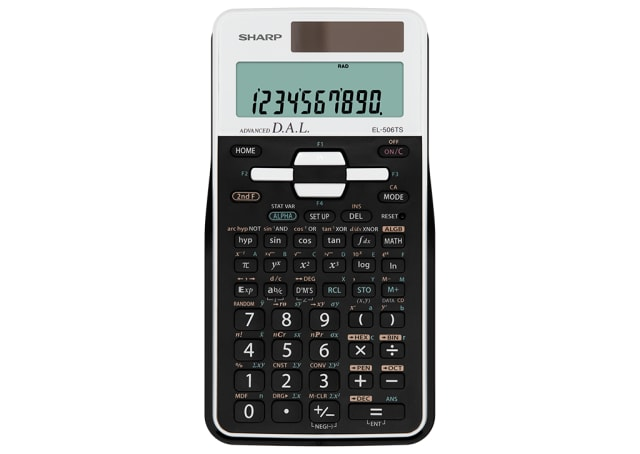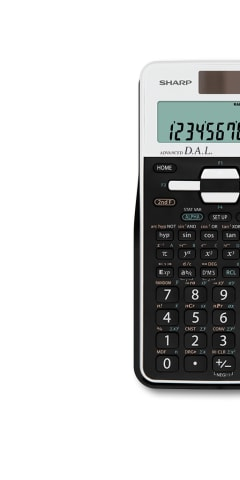EL506TSBWH

# Scientific Calculator With 470 Advance Functions - White

The EL506TSBWH performs over 470 advanced scientific functions and utilizes a 2-line display and Multi-Line Playback to make scientific equations easier for students to solve. It is ideal for students studying general math, algebra, geometry, trigonometry, and calculus. In addition, it can be used for statistics, biology, chemistry, and physics. Flexible, advanced, and despite its 470 functions still easy to use, this scientific calculator covers all the needs of daily school and university demands.

POA## Scientific Calculator## LCD Display## Twin Powered## Fraction Calculation• A scientific calculator designed to calculate problems in science, engineering, and mathematics. Great for school or engineers.
• Two-line display showing equation entered on top line, and answer on the bottom line allowing users to check both the equation and answer at the same time. User can amend the equation if needed.
• Solar powered with 1 x LR44 battery as backup, calculator can be used both during the day and night. Battery is secured with child-safe lock. Operating time is approximately 5000 hours, using the alkaline battery only. Auto power off after approximately 10 minutes.
• 9 values can be stored in calculator, with 8 temporary memories and 1 independent memory.
• Can perform 470 advanced scientific functions.
• Converting decimal to scientific or engineering notation.
• Allows you to enter numbers and equations as fractions.
• In addition to the four basic arithmetic functions (excluding division between matrices), X3, X2, and X-1, users are provided with transpose and cumulative matrix functions.
• Calculating complex numbers (in the form of a + bi).
• When a series of bivariate data has been entered correctly, then the calculator can be used to find the values of a and b, to give the equation of the line of regression from which we can make estimates of the variable y of the equation y=a+bx.
Calculator Types
Scientific Calculators
Display
2 Line LCD
Power Source
Solar cell / LR44 x 1
Memory
9
Scientific & Stats function
470
Fraction Key
Yes